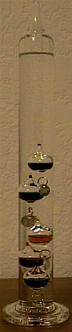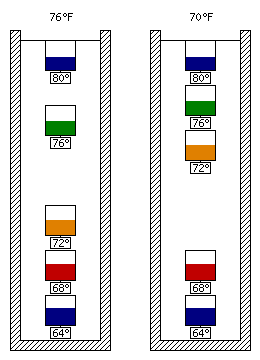# Galilean Thermometer

2005-01-04 - I have contributed most of the contents of this page to Wikipedia under the Galileo thermometer article.

In September 2000, I received as a birthday gift a fascinating type of thermometer invented by Galileo hundreds of years ago. Unlike most other types of thermometers, it is colourful and aesthetically pleasing.

## What Is It?

A galilean thermometer is a sealed vertical glass cylinder mostly filled with a clear liquid. Submerged in the liquid are several smaller glass bulbs, each having a tag indicating a particular temperature. As the temperature changes, the smaller glass bulbs will rise and sink (see How It Works below). The temperature is read by looking at tag attached to the lowest floating bulb.

## How It Works

The galilean thermometer works due to the principle of buoyancy. Buoyancy determines whether objects float or sink in a liquid, and is responsible for the fact that even boats made of steel can float (of course, a bar of steel by itself will sink).

The only factor that determines whether an object will float or sink in a particular liquid relates the object's mass to the mass of the liquid displaced by the object when submerged. If the object's mass is greater than the mass of liquid displaced, the object will sink. If the object's mass is less than the mass of liquid displaced, the object will float.Figure 1. (view source)

In the figure above, there are two objects, each a cube 10 cm by 10 cm by 10 cm (the figure is not to scale). The mass of water displaced by an object of this size is 1 kg. The brown object on the left is floating because the mass of water it is displacing (0.5 kg, half the object is submerged and half is above water) is equal to the mass of the object. The green object on the right has sunk because the mass of water it is displacing (1 kg) is less than the object's mass (2 kg).Figure 2. (view source)

Not all objects made of the green material above will sink. In figure 2, the interior of the green object has been hollowed out. The total mass of the object is now 0.5 kg, yet its volume remains the same, so it floats half way out of the water like the brown object in figure 1.

In the examples above, the liquid in which the objects have been floating is assumed to be water. Water has a density of 1 kg/L, which means that the volume of water displaced by any of the above objects is 1 kg.

Galileo discovered that the density of liquids varies slightly with temperature. As the temperature increases, the density of the liquid decreases. This is the key to how the galilean thermometer works.Figure 3. (view source)

Figure 3 shows a 1 kg hollow object made of the green material. In the left hand container, the density of the liquid is 1.001 kg/L. Since the object weighs less than the mass of water it displaces, it floats. In the right hand container, the density of the liquid is 0.999 kg/L. Since the object weighs more than the mass of water it displaces, it sinks. This shows that very small changes in the density of the liquid can easily cause an almost-floating object to sink.

In the galilean thermometer, the small glass bulbs are partly filled with a different (coloured) liquid. Each is filled with a slightly different amount, ranging from lightest at the uppermost bulb to heaviest at the lowermost bulb. The clear liquid in which the bulbs are submerged is not water, but some inert hydrocarbon (probably chosen because its density varies with temperature more than water does).Figure 4. (view source)

Figure 4 shows a schematic representation of my thermometer at two different temperatures (the temperature markings on my thermometer are in Fahrenheit).